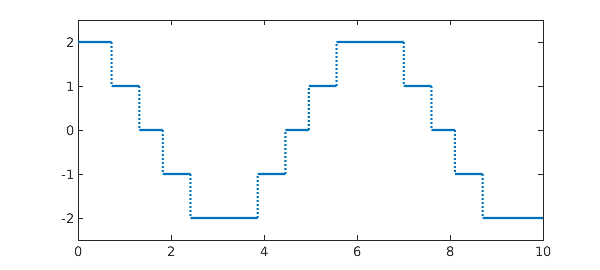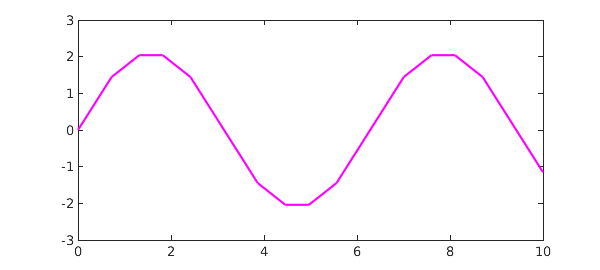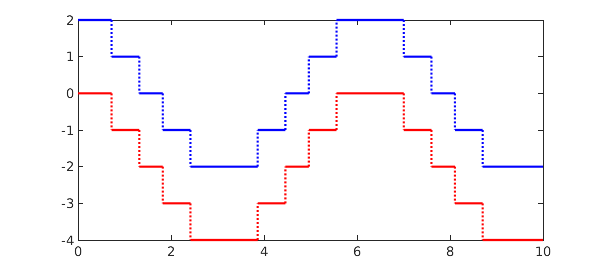Suppose we have a function, like this one:

x = chebfun('x',[0 10]);
f = round(2*cos(x));
plot(f), ylim(2.5*[-1 1])The Chebfun command sum returns the definite integral over the prescribed interval, which is just a number:

format long, sum(f)
ans =
-1.150444078461245


You can also calculate the definite interval over a subinterval by giving two additional arguments, like this:

sum(f,3,4)
ans =
-1.864326901403210


To compute an indefinite integral, use the Chebfun command cumsum. This returns a chebfun defined over the given interval:

g = cumsum(f);
plot(g,'m')Thus another way to compute the integral over a subinterval would be to take the difference of two values of the cumsum:

g(4) - g(3)
ans =
-1.864326901403210


As always in calculus, when working with indefinite integrals you must be careful to remember the arbitrary constant that may be added. Thus for example, if you integrate $f$ and then differentiate it, you get $f$ back again:

norm( diff(cumsum(f)) - f )
ans =
0


If you differentiate $f$ and then integrate it, on the other hand, you get something different:

norm( cumsum(diff(f)) - f )
ans =
6.324555320336759


Plotting the two instantly alerts us that we forgot to add back in the value at the left endpoint, namely $f(0) = 2$:

plot(f,'b',cumsum(diff(f)),'r')Sure enough, adding this number makes the two functions agree:

norm( f(0)+cumsum(diff(f)) - f)
ans =
0